# NumPy Array manipulation: rollaxis() function

## numpy.rollaxis() function

The rollaxis() function is used to roll the specified axis backwards, until it lies in a given position.
This function continues to be supported for backward compatibility, but you should prefer moveaxis. The moveaxis function was added in NumPy 1.11.

Syntax:

`numpy.rollaxis(a, source, destination)`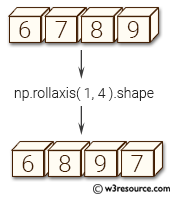Version: 1.15.0

Parameter:

Name Description Required /
Optional
a Input array. Required
axis The axis to roll backwards. The positions of the other axes do not change relative to one another. Required
start The axis is rolled until it lies before this position. The default, 0, results in a "complete" roll. Optional

Return value:

res [ndarray] For NumPy >= 1.10.0 a view of a is always returned. For earlier NumPy versions a view of a is returned only if the order of the axes is changed, otherwise the input array is returned.

Example-1: numpy.rollaxis() function

``````>>> import numpy as np
>>> a = np.ones((2,3, 4, 5))
>>> np.rollaxis(a, 2, 1).shape
(2, 4, 3, 5)
``````

Pictorial Presentation: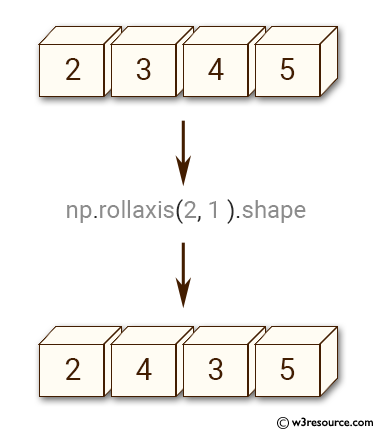Example-2: numpy.rollaxis() function

``````>>> import numpy as np
>>> y = np.ones((2,3, 4, 5))
>>> np.rollaxis(y, 2, 1).shape
(2, 4, 3, 5)
``````

Pictorial Presentation: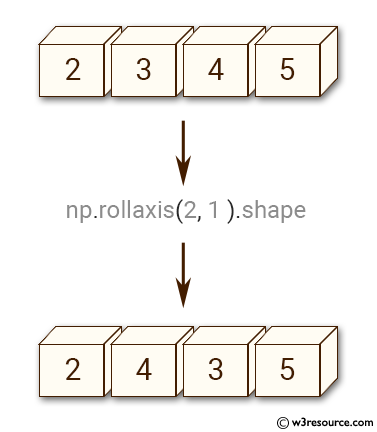Example-3: numpy.rollaxis() function

``````>>> import numpy as np
>>> y = np.ones((2,3, 4, 5))
>>> np.rollaxis(y, 3).shape
(5, 2, 3, 4)
``````

Pictorial Presentation: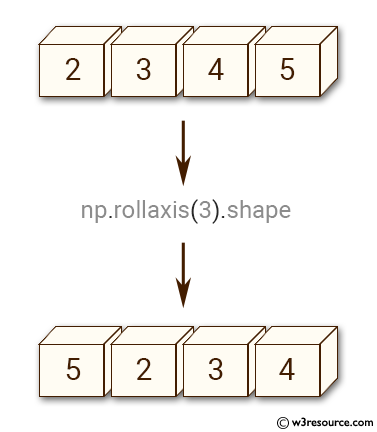Example-4: numpy.rollaxis() function

``````>>> import numpy as np
>>> y = np.ones((2,3, 4, 5))
>>> np.rollaxis(y, 1, 3).shape
(2, 4, 3, 5)
``````

Pictorial Presentation: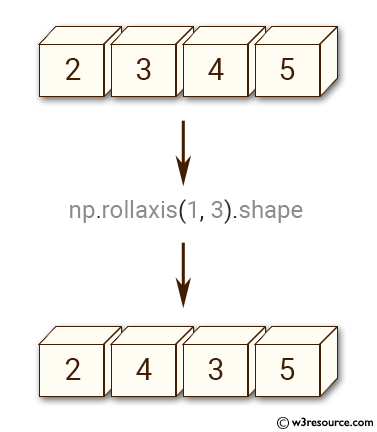Python - NumPy Code Editor:

Previous: moveaxis()
Next: swapaxes()

﻿